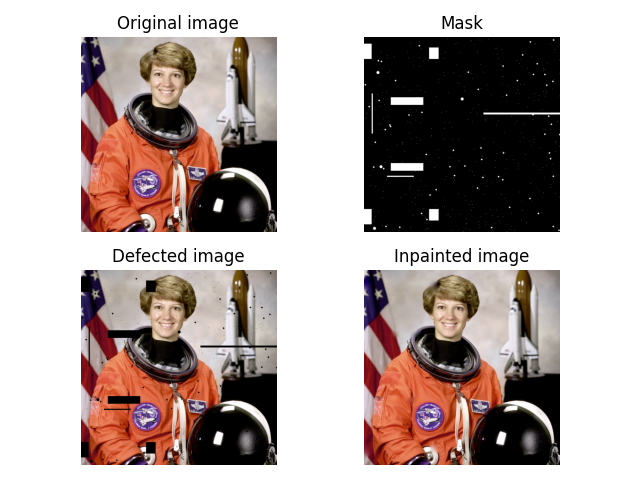# Inpainting#

Inpainting  is the process of reconstructing lost or deteriorated parts of images and videos.

The reconstruction is supposed to be performed in fully automatic way by exploiting the information presented in non-damaged regions.

In this example, we show how the masked pixels get inpainted by inpainting algorithm based on ‘biharmonic equation’-assumption   .```import numpy as np
import matplotlib.pyplot as plt

from skimage import data
from skimage.morphology import disk, binary_dilation
from skimage.restoration import inpaint

image_orig = data.astronaut()

# Create mask with six block defect regions

# add a few long, narrow defects

# add randomly positioned small point-like defects
rstate = np.random.default_rng(0)
for radius in [0, 2, 4]:
# larger defects are less common
thresh = 3 + 0.25 * radius  # make larger defects less common

# Apply defect mask to the image over the same region in each color channel
image_defect = image_orig * ~mask[..., np.newaxis]

fig, axes = plt.subplots(ncols=2, nrows=2)
ax = axes.ravel()

ax.set_title('Original image')
ax.imshow(image_orig)

ax.set_title('Defected image')
ax.imshow(image_defect)

ax.set_title('Inpainted image')
ax.imshow(image_result)

for a in ax:
a.axis('off')

fig.tight_layout()
plt.show()
```

Total running time of the script: ( 0 minutes 0.588 seconds)

Gallery generated by Sphinx-Gallery